# Fractional Numbers

## NUMBERS AND OPERATIONSA fractional number or fraction expresses a certain number of portions that are taken from a whole divided in equal parts.

It is represented by a dividing bar (oblique or horizontal), also called the "fraction line" that separates the first quantity (numerator) from the second (denominator).

#### Recognize the terms of a fraction

Do you know what a fraction is? And could you explain what the numerator and denominator represent? Don't worry, we offer you the explanation, an interactive game and some worksheets in PDF for you to practice and understand easily.

SPANISHENGLISHWe define the concept of fraction as "the number obtained by dividing a natural number (positive integer) into equal parts"

ELEMENTS OF A FRACTION

- The numerator (top number) of a fraction indicates the number of parts that are chosen from the unit.

- The denominator (bottom number) represents the number of equal parts the unit is divided into.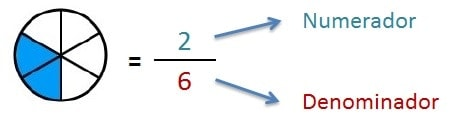#### Read and write simple fractions

We present you some printable activities accompanied by their explanation and an educational game so that you can learn to read and write any fraction.

SPANISHENGLISHHOW TO READ AND WRITE FRACTIONS

To correctly read and write any fraction, initially, we will read the numerator and then the denominator:

- The numerator is read and written using the cardinal numbers or natural numbers: one, two, three, four, five, thirty-six, hundred fifteen, nine hundred and eighty four...

- The denominator is read and written using the partitive numbers:

2 = half / halves

3 = third/s

4 = quarter/s

5 = fifth/s

6 = sixth/s

7 = seventh/s

8 = eighth/s

9 = ninth/s

10 = tenth/s

We present a visual scheme to facilitate the assimilation of learning to read and write fractional numbers: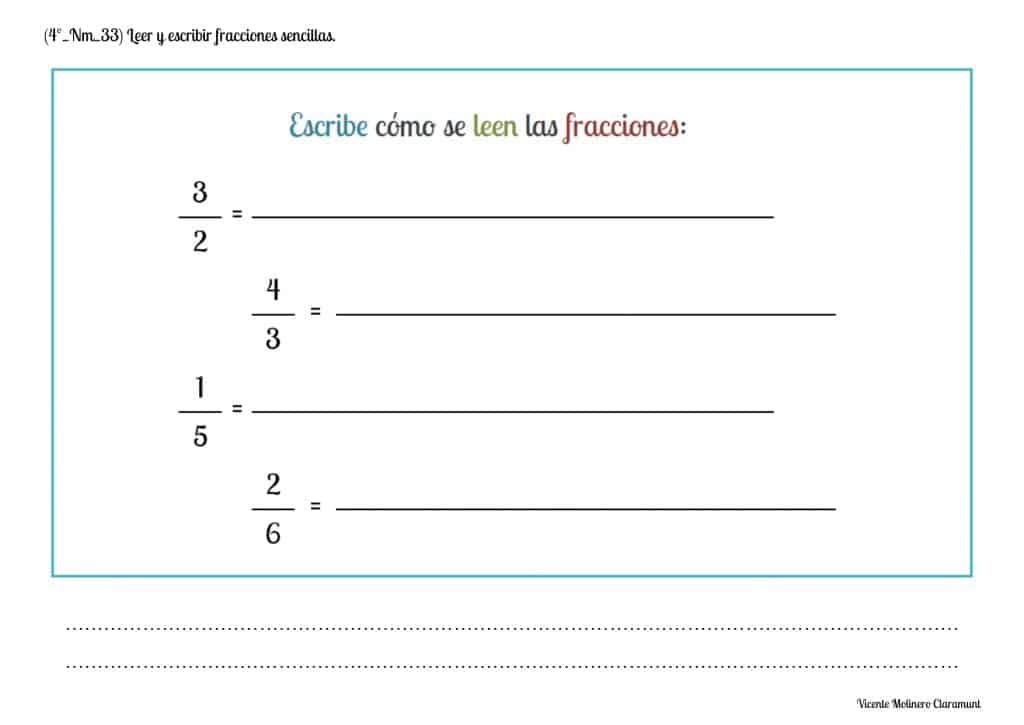#### Interpret fractions as dividing two whole numbers

Every fraction of two natural numbers (positive integers) always represents the division between these numbers.

To express the quotient of a fraction (also called "result"), we will divide the numerator by the denominator:#### Interpret and graph fractions

FRACTIONS LESS THAN ONE

We teach you to graphically represent fractions less than one, that is, those fractions in which the numerator is less than the denominator.

SPANISHENGLISHNUMERATOR < DENOMINATOR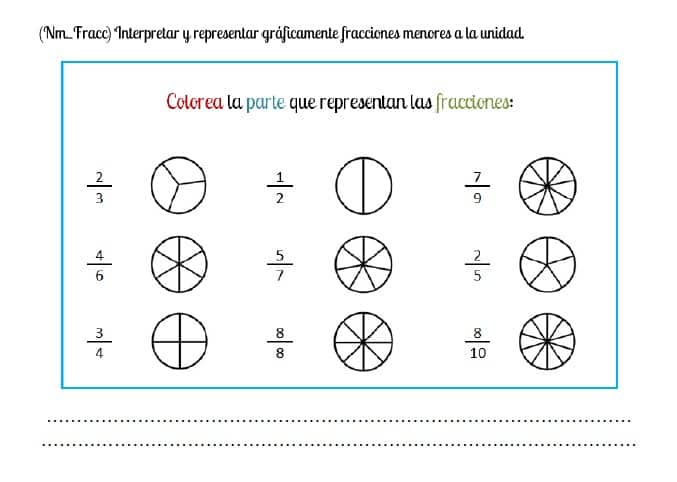FRACTIONS GREATER THAN ONE

We show you how to graphically represent fractions greater than one, that is, those fractions in which the numerator is greater than the denominator.

SPANISHENGLISHNUMERATOR > DENOMINATOR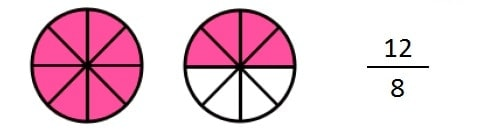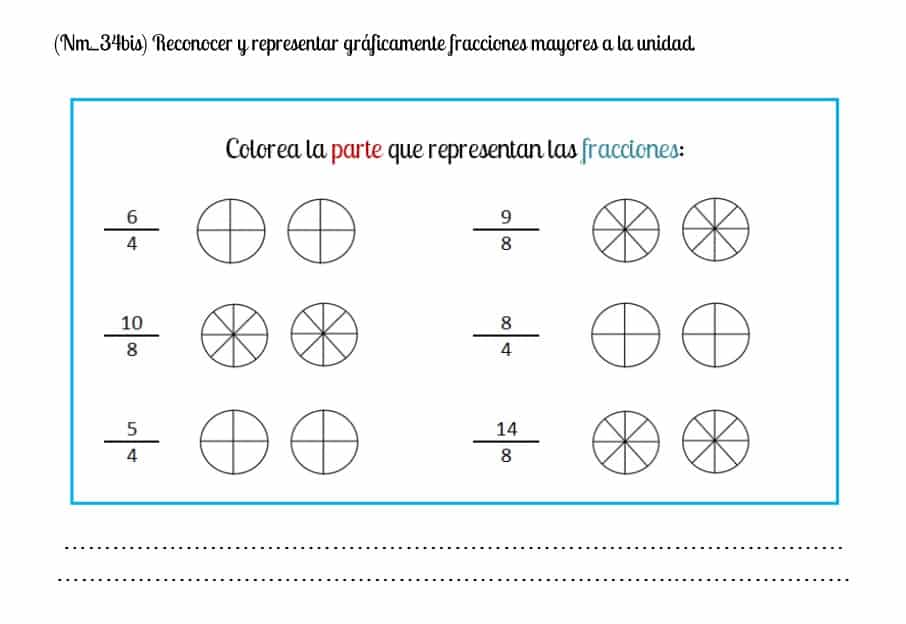#### Compare fractions of the same denominator (like fractions) with each other

When comparing different fractions with the same denominator (also called "like fractions") with each other, the fraction with the greater numerator will be greater.

View the lesson to understand it in a simple way and practice with the activities in PDF using great than (>), less than (<) and equal to (=) signs.

SPANISHENGLISHIn the following example, the fraction 5/9 will be greater than 4/9 because its numerator is greater ( 5 > 4 ):#### Compare fractions of the same numerator with each other

When comparing different fractions with the same numerator (also known as "same numerator fractions") with each other, the fraction with lower denominator will be greater.

View the lesson for quick understanding and solve our activities to keep improving your fraction comparisons.

SPANISHENGLISHIn the lesson that we propose, the fraction 1/2 will be greater than 1/4 because its denominator is smaller ( 2 < 4 ):#### Recognize and interpret equivalent fractions through their graphic representation

We teach you how to recognize and interpret when two fractions are equivalent to each other through their graphical representation to make it easier for you to learn. Remember that two or more fractions will be equivalent if, even though their numerator and denominator are different, they represent the same portion of the unit.

In the proposed example, you can see that the fractions 1/3 and 2/6 represent graphically the same part of the unit (equivalent portions):

Therefore, it will be the same to eat one-third of a cake as two-sixths of the same cake.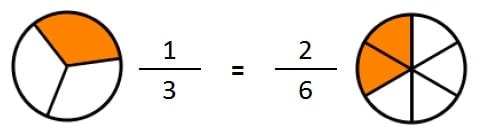#### Adding fractions of the same denominator (like fractions) by graphing

We show you how to solve the addition of fractions with the same denominator using their graphical representation. Remember, we will only add the numerators of each fraction and keep the denominator common.

SPANISHENGLISH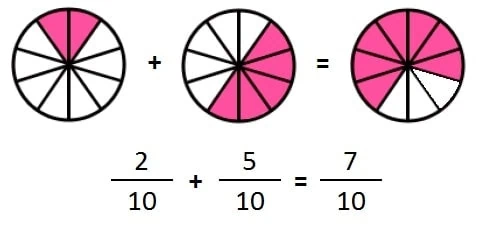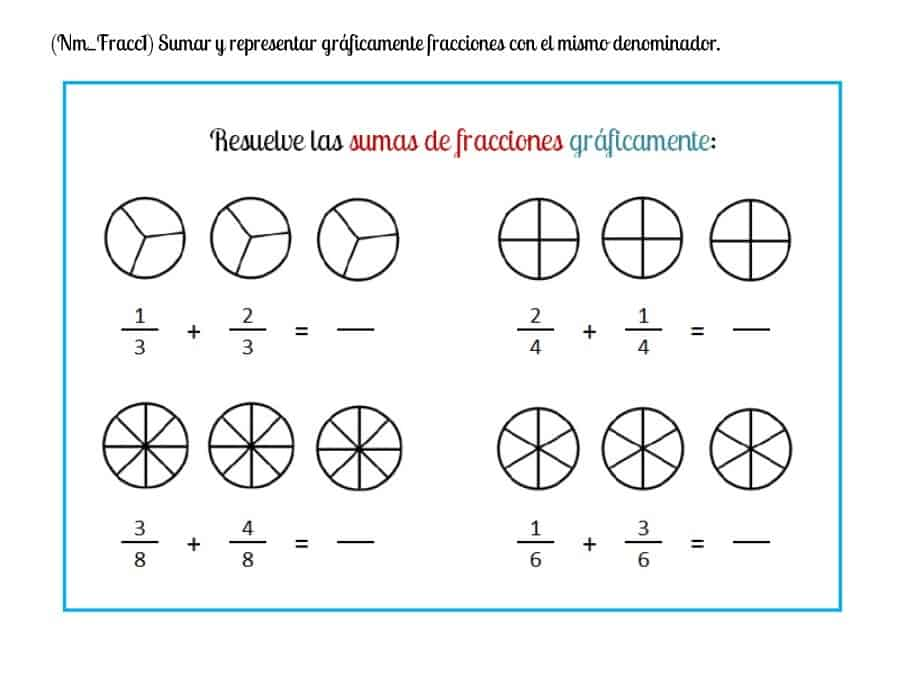#### Add fractions of the same denominator (like fractions)

To solve any sum of fractions with the same denominator, we will add the numerators of each fraction leaving the same denominator that they have in common.

SPANISHENGLISH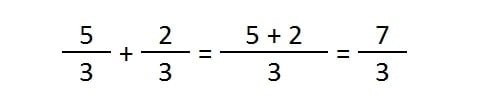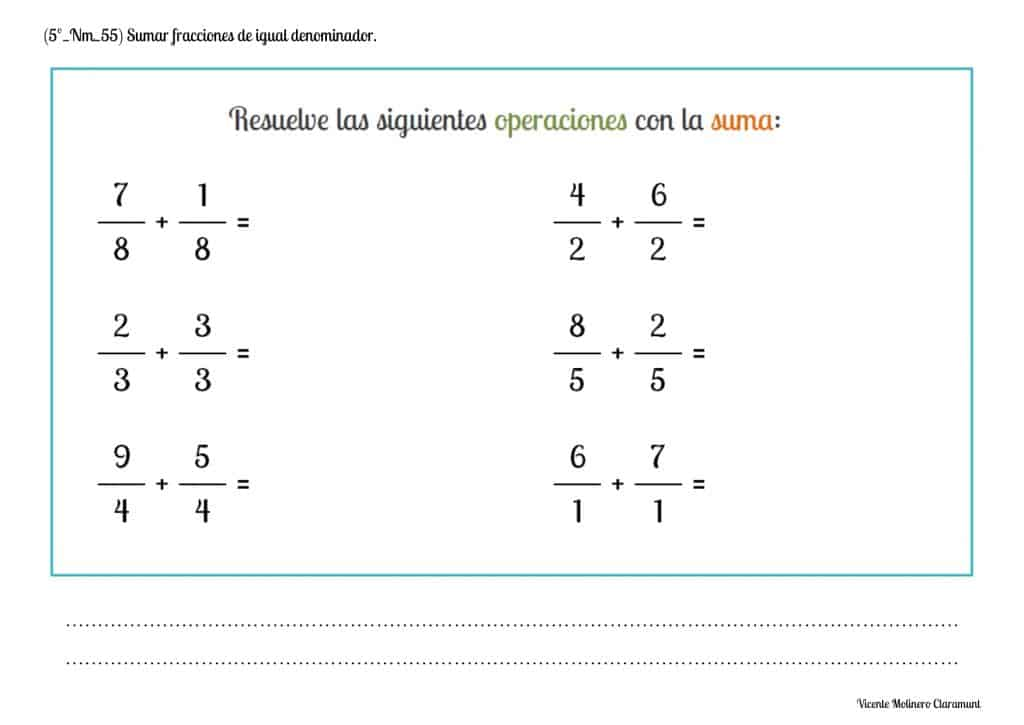#### Subtracting fractions of the same denominator (like fractions) by graphing

We show you how to solve the subtraction of fractions with the same denominator using their graphical representation. Remember, we will only subtract the numerators from each fraction and keep the denominator common.

SPANISHENGLISH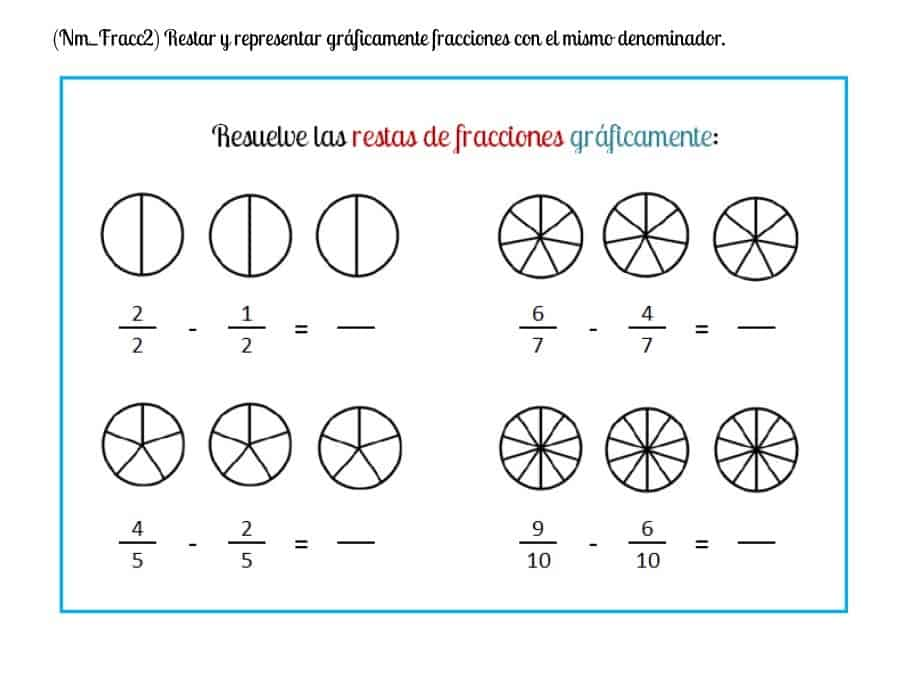#### Subtract fractions of the same denominator (like fractions)

To solve any subtraction of fractions with the same denominator, we will subtract the numerators of each fraction leaving the same denominator that they have in common.

SPANISHENGLISH#### Read and write decimal fractions

Do you know what a decimal fraction is? And would you know how to represent it ?

We define the concept of "decimal fraction" as that fractional number in which the denominator is a power of base ten, for example, 10, 100, 1,000, 10,000, etc.

Here's how decimal fractions are read and written based on the power of base ten in the denominator:

2/10 = 2 Tenths

6/100 = 6 Hundredths

9/1.000 = 9 Thousandths

Here is a lesson, an educational game and some exercises in PDF for you to learn and practice.

SPANISHENGLISH#### Recognize and graph decimal fractions

Can you recognize the portion that represents a decimal fraction? We have put together a set of tasks for you to practice graphing easily.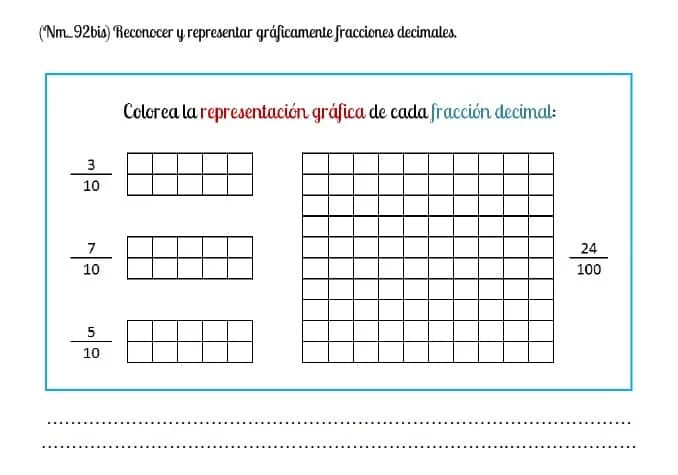Select more exercises, worksheets and activities of Mathematics for each of the 4 learning blocks of the Educational Curriculum ("Numbers and Operations", "Quantities and Measurements", "Geometric and Spatial Reasoning" and "Data Analysis and Probability") aimed at improving the logical-mathematical competencies and skills that are developed throughout "Grade 3" or "Year 4":